﻿ Vibration of Visco-Elastic Parallelogram Plate with Thickness Variation Linearly in one Direction and Parabolic in another Direction

### Vibration of Visco-Elastic Parallelogram Plate with Thickness Variation Linearly in one Direction an...

Arun Kumar Gupta, Anuj KumarOPEN ACCESSPEER-REVIEWED

## Vibration of Visco-Elastic Parallelogram Plate with Thickness Variation Linearly in one Direction and Parabolic in another Direction

Arun Kumar Gupta1,, Anuj Kumar1

1Department of Mathematics, M. S. College, Saharanpur, U.P., India

### Abstract

The main objective of the present investigation is to study the vibration of visco-elastic parallelogram plate whose thickness varies bi-directionally. It is assumed that the plate is clamped on all the four edges and that the thickness varies linearly in one direction and parabolically in another direction. Using the separation of variables method and Rayleigh-Ritz technique with a two-term deflection function, the governing differential equation has been solved for vibration of visco-elastic parallelogram plate. For visco-elastic, the basic elastic and viscous elements are combined. We have taken Kelvin model for visco-elasticity that is the combination of the elastic and viscous elements in parallel. Here the elastic element means the spring and the viscous element means the dashpot. The assumption of small deflection is made. Visco-elastic of the plate is taken of the “Kelvin Type”. Time period and deflection function at different point for the first two modes of vibration are calculated for various values of taper constant, aspect ratio and skew angle and results are presented in tabular form. Alloy “Duralumin” is considered for all the material constants used in numerical calculations.

• Gupta, Arun Kumar, and Anuj Kumar. "Vibration of Visco-Elastic Parallelogram Plate with Thickness Variation Linearly in one Direction and Parabolic in another Direction." American Journal of Applied Mathematics and Statistics 1.5 (2013): 103-109.
• Gupta, A. K. , & Kumar, A. (2013). Vibration of Visco-Elastic Parallelogram Plate with Thickness Variation Linearly in one Direction and Parabolic in another Direction. American Journal of Applied Mathematics and Statistics, 1(5), 103-109.
• Gupta, Arun Kumar, and Anuj Kumar. "Vibration of Visco-Elastic Parallelogram Plate with Thickness Variation Linearly in one Direction and Parabolic in another Direction." American Journal of Applied Mathematics and Statistics 1, no. 5 (2013): 103-109.

 Import into BibTeX Import into EndNote Import into RefMan Import into RefWorks

### 1. Introduction

Leissa [1, 2, 3] discussed the recent studies in plate vibration. Sobotka  used analysis of thick visco-elastic plates. The theory of linear visco-elasticity has been discussed by Bland . Ilanko  solved comments on the historical bases of the Rayleigh and Ritz methods. Eslami et al.  discussed coupled thermo elasticity of shell of revolution effect of normal stress and coupling. Leissa and Narita  discussed vibration studies for simply supported symmetrically laminated rectangular plates.

Lekhnitski  analyzed anisotropic plates. Zhang and Zu  solved non-linear vibrations of visco-elastic moving belts part-I: force vibration analysis. Zhang and Zu  used non linear vibration of parametrically excited visco-elastic moving belts part-II stability analysis. Mivhel  solved a periodic problem in visco-elasticity with variable coefficients. The survey of aero thermo-elasticity is discussed by Garrick . Gnossi and Laura  have discussed transverse vibrations of rectangular orthotropic plates with one or two free edges while the remaining is elastically restrained against rotation. Park and Mongeau  used the vibration and radiation of visco elastically supported midline plates. Gupta et al.  study the vibration of visco-elastic parallelogram plate of linearly varying thickness.

A simple model presented here is to study the effect of linearly thickness variation in one direction and parabolic in another direction on vibration of visco-elastic parallelogram plate having clamped boundary conditions on all the four edges. The hypothesis of small deflection and linear visco-elastic properties are made. Using the separation of variables method, the governing differential equation has been solved for vibration of visco-elastic parallelogram plate. An approximate but quite convenient frequency equation is derived by using Rayleigh-Ritz technique with a two-term deflection function. It is assumed that the visco-elastic of the plate is of the “Kelvin Type”. Time period and deflection function at different point for the first two modes of vibration are calculated for various values of taper constant, aspect ratio, and skew angle and results are presented in tabular and graphically form.

### 2. Equation of Transverse Motion

The maximum kinetic energy, Tmax, and the maximum strain energy, V in the plate when it is executing transverse vibration mode shape W(ξ, η) in skew coordinates are :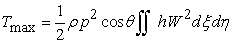(1)

andor(2)

and(3)

A comma followed by a suffix denotes partial differential with respect to that variable. Here p2 is a constant.

Here solution w(ξ, η ,t)can be taken in the form of products of two functions as for free transverse vibration of the parallelogram plate so that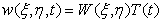(4)

where T(t) is the time function and W is the maximum displacement with respect to time t.

Assuming thickness variation of parallelogram plate linearly in ξ-direction and parabolically in η-direction, as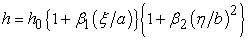(5)

where β1 and β2 is the taper constants in ξ and η- directions respectively and h0=h |ξ=η = 0.

The flexural rigidity of the plate can now be written as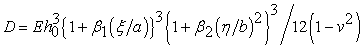(6)

### 3. Solution and Frequency Equation

In using the Rayleigh-Ritz technique, one requires maximum strain energy be equal to the maximum kinetic energy. So it is necessary for the problem consider here that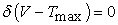(7)

for arbitrary variations of W satisfying relevant geometrical boundary conditions.

For a parallelogram plate, clamped (c) along all the four edges, the boundary conditions are(8)

and the corresponding two-term deflection function is taken as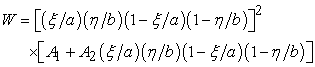(9)

Using equation (5) and (6) in equations (1) and (2) one obtains(10)

and(11)

Using eqs.(10) and (11) in eq.(7), one obtains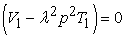(12)

where(13)

and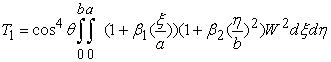(14)

Here,(15)

is a constant.

But equation (12) involves the unknown A1 and A2 arising due to the substitution of W(ξ, η) from eq (9). These two constants are to be determined from eq (12), as follows:(16)

Equation (16) simplifies to the form(17)

where bn1, bn2 (n = 1, 2 ) involve parametric constants and the frequency parameter.

For a non-trivial solution, the determinant of the coefficient of equation (17) must be zero. So one gets the frequency equation as(18)

where,

b11 = (F1- λ2 p2 B1),

b12=b21= (F2 – λ2 p2 B2),

and b22 =( F3 – λ2 p2 B3)

Here F1, F2, F3, B1, B2, B3 involves parametric constants, skew angle and aspect ratio.

From eq. (18), one can obtains a quadratic equation in p2 from which the two values of p2 can found. After determining A1 & A2 from eq.(17) , one can obtain deflection function W. Choosing A1=1, one obtains A2=(-b11/ b12) and then W comes out as(19)

### 4. Differential Equation of Time Function and its Solution

Time functions of free vibrations of visco-elastic plates are defined by the general ordinary differential equation (3). Their form depends on the visco-elastic operator Ď.

For Kelvin ,s model, one has(20)

where, ň is visco-elastic constant and G is shear modulus.

The governing differential equation of time function of an parallelogram plate of variable thickness, by using eq.(20)in eq.(3), one obtains(21)

Eq. (21) is a differential equation of order two for time function T.

Solution of eq.(21) comes out as(22)

where,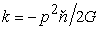(23)

and(24)

Let us take initial conditions as(25)

Using initial conditions from eq.(25) in eq.(22),one obtains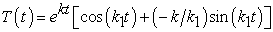(26)

Thus, deflection w may be expressed, by using eq.(26) and (19) in eq.(4), to give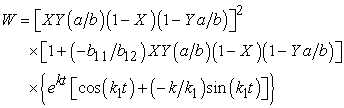(27)

Time period of vibration of the plate is given by(28)

where p is frequency given by eq.(18).

### 5. Results and Discussion

Time period and deflection are computed for visco-elastic parallelogram plate whose thickness varies linearly in one direction and parabolically in another direction for different value of skew angle(θ), taper constants (β1 and β2 ), and aspect ratio(a/b) at different points for first two mode of vibration. The material parameters have been taken as : E=7.08x1010N/M2, G=2.682x1010N/M2,

ň=1.4612x106N.S/M2, ρ=2.80x103Kg/M3, ν=.345 and h0=0.01 meter.

All the results are presented in the tables 1-21 .The value of time period (K) for β1=0.4, β2 =0.6 and θ=450 have been found to decrease 36.4152960% for first mode and 35.4467079% for second mode in comparison to rectangular plate at fixed aspect ratio (a/b=1.5).

The value of time period (K) for β1=0.4, β2 =0.6 and θ=450 have been found to decrease 30.2696427% for first mode and 29.7142383% for second mode in comparison to parallelogram plate of uniform thickness at fixed aspect ratio (a/b=1.5).

Table 1 show the results of time period(K) for different values of taper constant (β1) and fixed aspect ratio (a/b=1.5) for different values of skew angle (θ) and taper constant (β2) i.e. β2=0.0,θ=00; β2=0.0,θ=450; β2=0.6,θ=00; and β2=0.6,θ=450 for first two mode of vibration. It can be seen that the time period (K) decrease when taper constant( β1) increase for the first two mode of vibration .

Table 2 show the results of time period(K) for different values of values of skew angle (θ) and fixed aspect ratio (a/b=1.5) for values taper constant (β1 and β2) i.e. β1=0.0,β2=0.0; β1=0.4,β2=0.0; β1=0.0,β2=0.6; and β1=0.4,β2=0.6 for first two mode of vibration. It can be seen that the time period (K) decrease when skew angle (θ) increase for two mode of vibration. Table 3 show the results of time period(K) for different values of taper constant (β2) and fixed aspect ratio (a/b=1.5) for values of skew angle (θ) and taper constant (β1) i.e. β1=0.0,θ=00; β1=0.0,θ=450; β1=0.6,θ=00;and β1=0.6,θ=450 for first two mode of vibration. It can be seen that the time period (K) decrease when taper constant (β2) increase for two mode of vibration.

Table 4 and Table 5 show the results of time period(K) for different values of values of aspect ratio (a/b) and fixed angle (θ=00 and 450 ) for values taper constant (β1 and β2) i.e. β1=0.0,β2=0.0; β1=0.4,β2=0.0; β1=0.0,β2=0.6; and β1=0.4,β2=0.6 for first two mode of vibration. It can be seen that the time period (K) decrease when aspect ratio (a/b) increase for two mode of vibration.

#### Table 5. Time period K (in seconds) for different aspect ratio (a/b) and angle(θ=450 )

The value of deflection (w) for β1=0.4, β2 =0.6 and θ=450 have been found to increase 5.2670080% for first mode and no effect for second mode in comparison to parallelogram plate of uniform thickness for initial time 0.K at X=0.2, Y=0.4 and aspect ratio(a/b=1.5).

The value of deflection (w) for β1=0.4, β2 =0.6 and θ=450 have been found to decrease 2.7045301% for first mode and 0.2659574% for second mode in comparison to rectangular plate for initial time 0.K at X=0.2, Y=0.4 and a/b=1.5.

The value of deflection (w) for β1=0.4, β2 =0.6 and θ=450 have been found to increase 1.8912530% for first mode and decrease 10.9929078% for second mode in comparison to parallelogram plate of uniform thickness for time 5.K at X=0.2, Y=0.4 and a/b=1.5.

The value of deflection (w) for β1=0.4, β2 =0.6 and θ=450 have been found to decrease 6.3721941% for first mode and 13.4482759% for second mode in comparison to rectangular plate for time 5.K at X=0.2, Y=0.4 and a/b=1.5.

Tables 6 - 21 shows the results of deflection (w) for different values of X , Y and fixed taper constant (β1 =0.0,0.4 and β2 =0.0,0.6) , and aspect ratio (a/b=1.5) for two values of angle (θ) i.e. θ=00 and θ=450 for first two mode of vibration with time 0.K and 5.K. It can be seen that deflection (w) start from zero to increase then decrease to zero for first two mode of vibration (except second mode at Y= 0.2 and 0.4) and second mode of vibration deflection (w) at Y=0.2 start zero to increase then decrease then increase then decrease and finally become to zero and second mode of vibration deflection (w) at Y=0.4 start zero to decrease then increase and finally become to zero for different value of X.

### References

  Leissa A. W., Vibration of Plate, NASA SP-160, (1969).In article  Leissa A. W., Plate Vibration Research, 1976-1980, Classical Theory, The Shock and Vibration Digest, 13(9), (1981), 11-22.In article CrossRef  Leissa A. W., Recent Studies in Plate Vibrations: Part I, Classical Theory, The Shock and Vibration Digest, 19(2), (1987),11-18.In article CrossRef  Sobotka Z., Analysis of thick visco-elastic plates, Theoretical and Applied Mechanics, Proceeding Vol. I. Publishing House of the Bulgarian Academy of Sciences, Sofia., (1971),379-386.In article  Bland D.R., The theory of linear visco-elasticity, Pergamon Press, (1960).In article  Ilanko S., Comments on the historical bases of the Rayleigh and Ritz methods, J. Sound and Vibration, 319( 1-2),(2009), 731-733.In article CrossRef  Eslami M. R., Shakeri M., Ohadi A.R. and Shiari B., Coupled thermo elasticity of shell of revolution effect of normal stress and coupling, AIAA Journal, 37(4), (1999), 496-512.In article CrossRef  Leissa A. W. and Narita Y., Vibration studies for simply supported symmetrically laminated rectangular plates, Composite Structures, 12,(1989), 113-132.In article CrossRef  Lekhnitski S.G., Anistropic plates, Ist Ed.English trans., Am.Iron and Steel Inst. (New York N.Y.) ( 1956).In article  Zhang L. and Zu J.W., Non-linear vibrations of visco-elastic moving belts part-I: force vibration analysis, J. Sound and Vibration, 216(1),(1998),75-91.In article CrossRef  Zhang L and Zu J.W., Non linear vibration of parametrically excited visco-elastic moving belts part-II stability analysis, J. Appl.Mach., Trans. ASME, 66(2), (1999), 403-409.In article CrossRef  Mivhel R., A periodic problem in visco-elasticity with variable coefficients, Int. J. of Engg. Sci., 19, (1981), 1145-1168.In article CrossRef  Garrick I.E., Survey of Aero-thermo-elasticity, J. Aerospace Engg.,22,(1963), 140-147.In article  Gnossi R. O. and Laura P. A. A., Transverse vibrations of rectangular orthotropic plates with one or two free edges while the remaining are elastically restrained against rotation, Ocean Engg.,6(5),(1979),527-539.In article CrossRef  Park J. and Mongeau Luc., Vibration and radiation of visco-elastically supported mindlin plates, J. Sound and Vibration, 318(4-5),(2008),1230-1249.In article CrossRef  Gupta A.K., Kumar A. and Gupta Y.K., Vibration study of visco-elastic parallelogram plate of linearly varying thickness, International Journal of Engineering and Interdisciplinary Mathematics, 2(1),(2010),1-9.In article  Nagaya K., Vibrations and dynamic response of visco-elastic plates on non-periodic elastic supports, J. Engg. for Industry, 99, (1977),404-409.In article CrossRef